# Equivalent Ratios Examples

#### Equivalent Ratios

In order to find Equivalent Ratios of any given ratio, we multiply or divide the numerator and denominator of the ratio by the same non zero number.  We would explain this concept with the help of certain Equivalent Ratios Examples

#### Equivalent Ratios Examples

Equivalent Ratios Example 1:

Find the Equivalent ratio of 2 : 5?

9/15, 5/2, 8/20, 6/15

Explanation:

On multiplying or dividing each term of a ratio by the same non zero number, we get a ratio equivalent to the given ratio

For, 8/20

Both numerator and denominator of given fraction is multiplied by same nonzero number i.e 4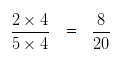8/20 is an equivalent ratio of 2/5

9/15 is not an equivalent ratio of 2/5. As both 2 and 5 are not multiply by same non zero number

5/2 is not an equivalent ratio of 2/5. As both 2 and 5 are not multiply by same non zero number

6/15 is not an equivalent ratio of 2/5 As both 2 and 5 are not multiply by same non zero number

Equivalent Ratios Example 2:

Find the Equivalent ratio of 4 : 3?

21/9, 20/15, 3/4, 20/9

Explanation:

On multiplying or dividing each term of a ratio by the same non zero number, we get a ratio equivalent to the given ratio

For, 20/15

Both numerator and denominator of given fraction is multiplied by same nonzero number i.e 5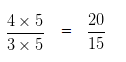20/15 is an equivalent ratio of 4/3

3/4 is not an equivalent ratio of 4/3. As both 4 and 3 are not multiply by same non zero number

21/9 is not an equivalent ratio of 4/3. As both 4 and 3 are not multiply by same non zero number

20/9 is not an equivalent ratio of 4/3. As both 4 and 3 are not multiply by same non zero number

Equivalent Ratios Example 3:

Find the Equivalent ratio of 2 : 3?

8/9, 3/2, 8/12, 4/9

Explanation:

On multiplying or dividing each term of a ratio by the same non zero number, we get a ratio equivalent to the given ratio

For, 8/12

Both numerator and denominator of given fraction is multiplied by same nonzero number i.e 4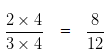8/12 is an equivalent ratio of 2/3

3/2 is not an equivalent ratio of 2/3. As both 2 and 3 are not multiply by same non zero number

8/9 is not an equivalent ratio of 2/3. As both 2 and 3 are not multiply by same non zero number

4/9 is not an equivalent ratio of 2/3. As both 2 and 3 are not multiply by same non zero number

Equivalent Ratios Example 4:

Find the Equivalent ratio of 5 : 4?

21/12, 4/5, 20/16, 18/12

Explanation:

On multiplying or dividing each term of a ratio by the same non zero number, we get a ratio equivalent to the given ratio

For, 20/16

Both numerator and denominator of given fraction is multiplied by same nonzero number i.e 4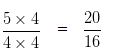20/16 is an equivalent ratio of 5/4

4/5 is not an equivalent ratio of 5/4. As both 5 and 4 are not multiply by same non zero number

21/12 is not an equivalent ratio of 5/4. As both 5 and 4 are not multiply by same non zero number

18/12 is not an equivalent ratio of 5/4. As both 5 and 4 are not multiply by same non zero number

Equivalent Ratios Example 5:

Find the Equivalent ratio of 5 : 6?

11/18 , 6/5, 15/24, 10/12

Explanation:

On multiplying or dividing each term of a ratio by the same non zero number, we get a ratio equivalent to the given ratio

For, 10/12

Both numerator and denominator of given fraction is multiplied by same nonzero number i.e 2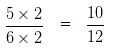10/12 is an equivalent ratio of 5/6

6/5 is not an equivalent ratio of 5/6. As both 5 and 6 are not multiply by same non zero number

11/18 is not an equivalent ratio of 5/6. As both 5 and 6 are not multiply by same non zero number

15/24 is not an equivalent ratio of 5/6. As both 5 and 6 are not multiply by same non zero number